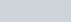The Titration Curve Paper

The Titration Curve (Revisited)

• The titration curve for soda ash (Na2CO3) is shown.
• An IMPORTANT POINT OF REFERENCE is given on the curve.

0

2

4

6

8

10

12

14

0

10

20

30

40

50

pH

Volume Titrant (mL)mL

18.50

1. Na2CO3 is soluble in water (as are ALL SODIUM COMPOUNDS). Write the ionic equation for the ionic equation for this process. If you don’t remember what this is look it up in any general chemistry textbook.

1. Once dissolved what happens to the sodium ions? Where do they go? Do they do anything?

1. Your ionic equation should show the carbonate ion. Write the chemical formula for carbonate. Is carbonate an acid or a base? Provide evidence of your answer using the titration curve. You must be specific in your answer. I want numbers and proof that carbonate is what you say it is.

1. Give an example of the substance that might be used as a titrant (give actual molecule used). What MUST be true about your chosen titrant in order for it to be suitable for a titration reaction?

1. Write the following chemical reactions:
• The base equilibrium reaction for carbonate in water
• The titration reaction when your chosen titrant reacts with carbonate in water. (NO Na+please!)

1. In the second reaction in part e) which species does your titrant actually react with, the analyte or something else? Explain.

1. Write the Henderson-Hasselbalch equation. That’s it. Just write the equation.

1. For a diprotic acid which value is bigger Ka1 or Ka2? Explain. What does the term pKa mean?

1. The Henderson-Hasselbalch equation gives the relationship between pH and pKa. Titration curves are written in terms of pH. Therefore pKa values are directly observable from the titration curve – even if the analyte is a base! (see your equation in part g)

• Where in the titration curve are pK values observable? Explain why this is so.
• What are the values of pKa1 and pKa2? (simple pH values please – 2, 4, 7, etc).
• Calculate the values of Ka1 and Ka2?

j)

• For a diprotic base which value is bigger Kb1 or Kb2?
• Using the values from part i) determine the values of pKb1 and pKb2
• Calculate the values of Kb1 and Kb2.

1. On a titration curve what is the significance of the steep rise or drop in pH (which ideally occurs with only a drop or two of titrant)? There are two such changes in this curve, at what volumes do they occur? (Give exact volumes)?

1. What volume of titrant (exact volume) is needed to completely neutralize the soda ash? Explain how you determined this number using specific values from the titration curve.

1. The principle species in solution refers to the principle form of the analyte.  What is the principle species at 0.00 mL? Explain.
• What is the volume of titrant (exact volume) needed so that [HCO3] = [CO32-]? Explain  What is the principle species at 18.50 mL? Explain.
• What is the volume of titrant (exact volume) needed so that [HCO3] = [H2CO3]? Explain  What is the principle species at 37.00 mL? Explain.

1. How do you calculate the pH when the principle species is HCO3? Calculate the pH based on the pKa values you gave in part i). Does this value match the expected pH as observed on the titration curve? (it should … if it doesn’t check your work)

1. What is the pH when the soda ash is stoichimetrically neutralized? Explain why this pH makes sense given that sodium carbonate is a base.

1. In the soda ash lab we used methyl purple as the indicator. Let’s assume the methyl purple is not available but the indicators we just used in lab (below) are available. Which indicator should you choose? Explain why your answer is the best choice and why the other two are not so good.
• methyl orange
• bromothymol blue
• phenolphthalein

1. Methyl purple is not on the provided indicator list (next page) but clearly must be OK or we would not have used it in the soda ash lab. Using the titration curve as evidence provide an estimated pKa for methyl purple. Explain your answer.